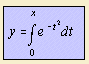# error function

## exponentialThe error function erf(x), usually normalized to one with a factor 2/√π, is the integral of the Gauss curve. It is also called the integrated Gauss function or the integrated distribution. The function gives the probability that a measurement under the influence of accidental errors has a distance less than x from the average value at the center.
The complementary error function erfc(x) is the same integral, but now from x to infinity.
For the generalized error function the upper as well as the lower integral boundary is a variable.
The error function is widely used in statistical calculations.
The curve resembles the cotangent.

The inverse error function is used in computing confidence intervals in statistics and in some algorithms for generating Gaussian random numbers.